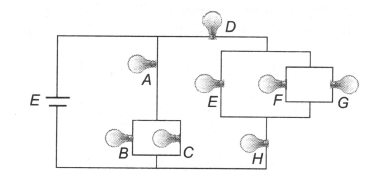Assuming all bulbs are identical, rank the brightnesses of the bulbs, from brightest to dimmest.

# Assuming all bulbs are identical, rank the brightnesses of the bulbs, from brightest to dimmest.1. A

A > D - H > B - C > E - F = G

2. B

A > B - C > D - H > E - F = G

3. C

A > D - H > E - F - G > B = C

4. D

All have equal brightness

Register to Get Free Mock Test and Study Material

+91

Verify OTP Code (required)

### Solution:

For the branch containing A, B and C.

${\mathrm{R}}_{eq}\text{\hspace{0.17em}}=\frac{3R}{2}$

Current in A : ${\mathrm{I}}_{1}=\frac{\mathrm{E}}{{\mathrm{R}}_{\mathrm{eq}}}\text{\hspace{0.17em}}=\text{\hspace{0.17em}\hspace{0.17em}}\frac{2\mathrm{E}}{3\mathrm{R}}$

Current in each of B and C = ${{I}^{\text{'}}}_{1}=\frac{{I}_{1}}{2}\text{\hspace{0.17em}}=\text{\hspace{0.17em}\hspace{0.17em}}\frac{E}{3R}$

For the branch containing D, E, F, G and H:

${\mathrm{R}}_{eq}\text{\hspace{0.17em}}=\frac{7R}{3}$

Current through D and H: ${I}_{2}=\frac{3E}{7R}$

Current through each of E, F and G:

${{\mathrm{I}}^{\text{'}}}_{1}=\frac{{\mathrm{I}}_{2}}{3}\text{\hspace{0.17em}}=\text{\hspace{0.17em}\hspace{0.17em}}\frac{\mathrm{E}}{7\mathrm{R}}\text{\hspace{0.17em}\hspace{0.17em}\hspace{0.17em}}\mathrm{Since}\text{\hspace{0.17em}\hspace{0.17em}}{\mathrm{I}}_{1}\text{\hspace{0.17em}\hspace{0.17em}}>\text{\hspace{0.17em}}{\mathrm{I}}_{2}\text{\hspace{0.17em}}>\text{\hspace{0.17em}\hspace{0.17em}}{{\mathrm{I}}^{\text{'}}}_{1}\text{\hspace{0.17em}}>\text{\hspace{0.17em}\hspace{0.17em}}{\mathrm{I}}^{\text{'}}$

A > D = H > B = C > E = F = GRegister to Get Free Mock Test and Study Material

+91

Verify OTP Code (required)